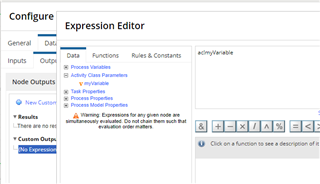# Saving Script Task Input Into Multiple Process Variables

Suppose in my process model, I want to calculate a value and save it into process models var1, var2 and var3.

Currently, I have used a script task to calculate this value three times, as input1, input2 and input3, then saving them respectively into var1, var2, var3.

Is there a nicer way to do this? I would have expected to be able to calculate the value once for input 1, and then save into variables var1, var2, var3 immediately.

Discussion posts and replies are publicly visible

• Hi Danny.

In script task ,calculate your value in ac ,and assign that ac value to var1, var2 and var3.

• Agreed with Krishna - to clarify a bit, what you would do is do your calculation in an input variable on the "Inputs" tab, and then in the "Outputs" tab, you would add 3 separate outputs, each one pointing to the PV you want to set (so "pv!var1", etc), and each one of them would be set to the input variable's value, i.e. "ac!myVariable".